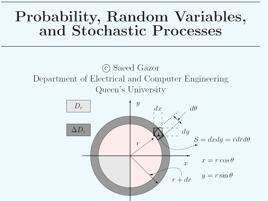3 afleveringen

The review of probability theory including probability spaces, random variables, probability distribution and density functions, characteristic functions, convergence of random sequences, and laws of large numbers. Fundamental concepts of random processes including stationarity, ergodicity, autocorrelation function and power spectral density, and transmission of random processes through linear systems. Special random processes, including Gaussian processes, with applications to electrical and computer engineering at a rigorous level.# ELEC-861 Probability, Random Variables and Stochastic Processes Queen's University

• Technologie

The review of probability theory including probability spaces, random variables, probability distribution and density functions, characteristic functions, convergence of random sequences, and laws of large numbers. Fundamental concepts of random processes including stationarity, ergodicity, autocorrelation function and power spectral density, and transmission of random processes through linear systems. Special random processes, including Gaussian processes, with applications to electrical and computer engineering at a rigorous level.